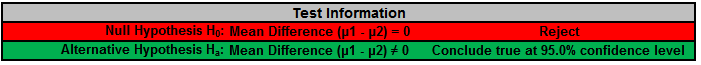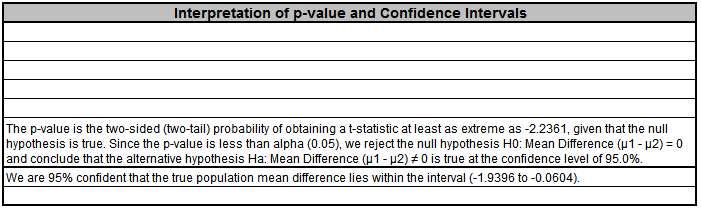Include Top

Basic Statistical Templates – 2 Sample t-Test and Confidence Interval (Compare 2 Means) Example

Click SigmaXL > Templates & Calculators > Basic Statistical Templates > 2 Sample t-Test & Confidence Interval (Compare 2 Means) to access the template.1. Enter summarized Sample Data, Null Hypothesis and Confidence Level in cells with yellow highlight. Do not modify any other part of this worksheet.
2. Select Alternative Hypothesis and "Assume Equal Variances" using drop-down.
3. This calculator will automatically detect the version of Excel and use the appropriate statistical functions. If Excel 2010 or higher, then the new Excel 2010 statistical functions are used.

# Web Demos

Our CTO and Co-Founder, John Noguera, regularly hosts free Web Demos featuring SigmaXL and DiscoverSim